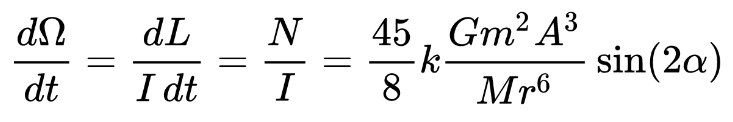# Cleaning Up Our Orbiting Debris Could Give Us All More Hours in a Day

There is a lot of debris left in space from our activities up there. It makes it very difficult to put satellites or astronauts into orbit. There is also the problem that there isn’t nearly enough time in the day to get everything done that we want to get done. What if I were to tell you that we can fix both of these seemingly disparate problems with one simple solution?

The angular momentum of a spinning system is invariant such that when its rotational velocity decreases, the distance increases, and vise versa. You may have seen this effect with ice skaters.

Thus, if we slow down the rotation of the earth, all of the debris orbiting the earth will move further away, like the ice skaters arms when she slows her rotation. And like the moon.So, if we slow the rotation down enough, it will send all that debris flying off into deep space, making it some extraterrestrial’s problem. Here’s how we do it:

We calculate the slowdown of earth thus:and using the ratio of mass density of ocean water to earth:

k = water/rock = 1 g/cm3 vs. 5.5 g/cm3 = 1.8

and use the moon’s bulge angle:

α = 1/13

we plug in the debris’ mass for m, debris distance from earth’s rotational axis for A, earth’s mass for M, and earth’s radius for r, we get

(45/8)(k){[Gm2A3]/[Mr6]}sin(2α)

=(45/8)(0.18){[(6.7×10-11 m3/kgs2)(8.4×106 kg)2(7.2×106 m)3]/[(6.0×1024 kg)(6.4×106 m)6]}sin(2/13)

= (0.0027){[3.4×1010]/[4.1×1065]}

= (2.7×10-3)(8.3×10-56) = (2.2×10-58)s-2

which means that the earth’s rotation about its axis is decelerating by 2.2×10-58 radians per second every second due to tidal forces caused by the space debris. At that rate, it will take

(7.3×10-5 rad/s) / (2.2×10-58 rad/s2) = 3.3×1053 seconds or 1046 years for the earth to slow to a stop and all the debris to go flying off.

But if we want to slow the earth’s 7.3×10-5 rad/sec rotation down to 0 rad/sec, we’ll need to use some energy. Why not use petroleum gas energy?

——————————-

We can get the rotational kinetic energy of the earth rotating on its axis with:

KErot = (Iω2)/2

where

I = moment of inertia: I = (2mr2)/5 for a uniform sphere (earth approximation)
m = the mass of the earth, which is 6.0×1024 kg
r = the distance from its pivot, which is the radius of the earth: 6.4×106 meters
ω = rotational velocity at the earth’s surface in radians/time, which is:

2π/24hr = 2π/86,400s =  7.3×10-5 rad/sec

So:

KErot = (Iω2)/2 = ([(2(6.0×1024 kg)(6.4×106 m)2)/5]⋅(7.3×10-5 rad/sec)2)/2

= [(1.5×1031)(5.3×10-9 rad/s2)]/2 = [8.1×1022 kgm2/s2]/2

=  4.0×1022 Joules

So we would need

4.0×1022 Joules / 1.25×108 Joules/gallon

= 3.3×1014 gallons of petroleum gas

And there are an estimated 1.5×1012 gallons of petroleum left on earth…which isn’t nearly enough. Oh well. I guess we’re stuck with the junk… in SPACE!